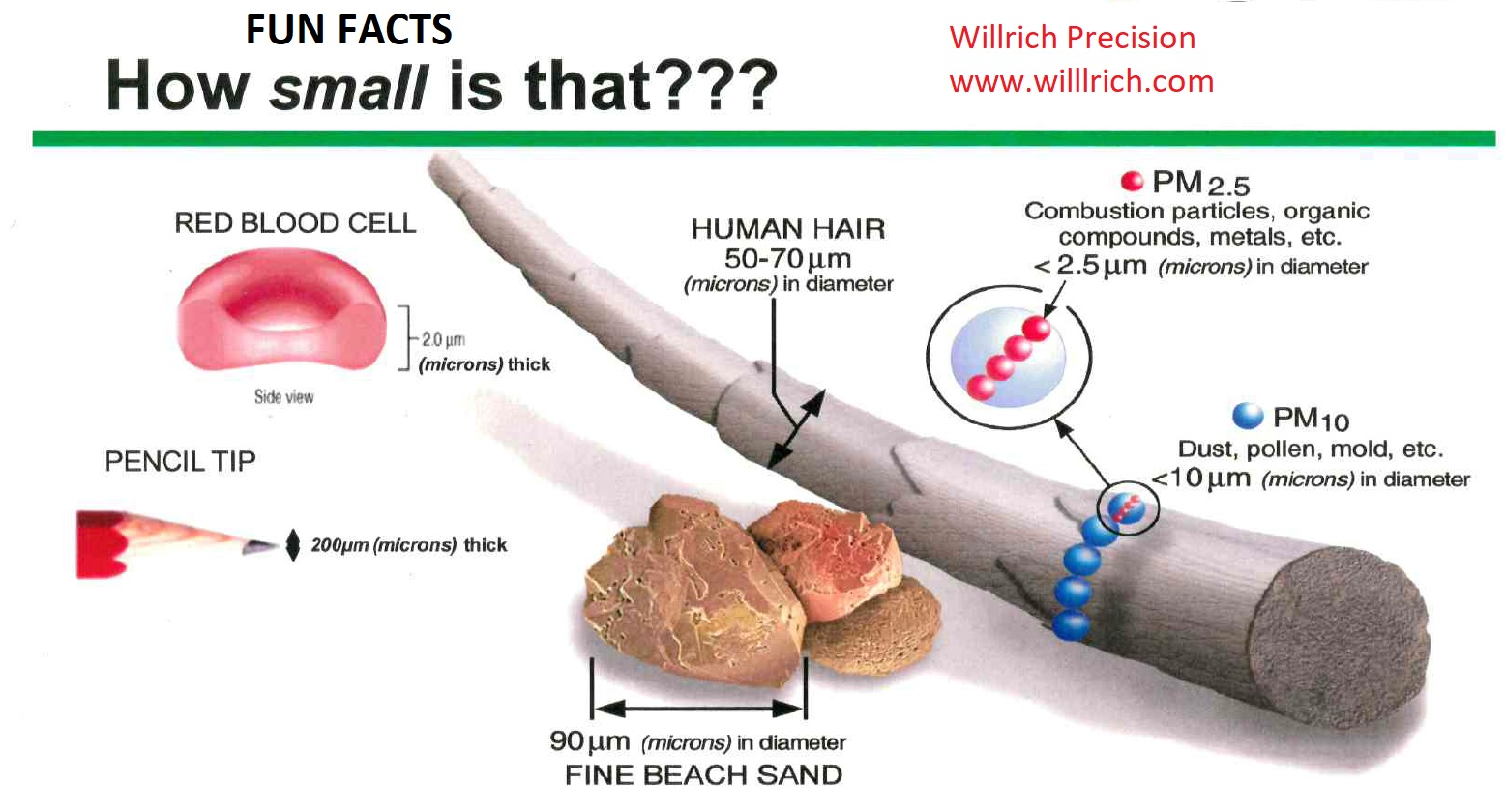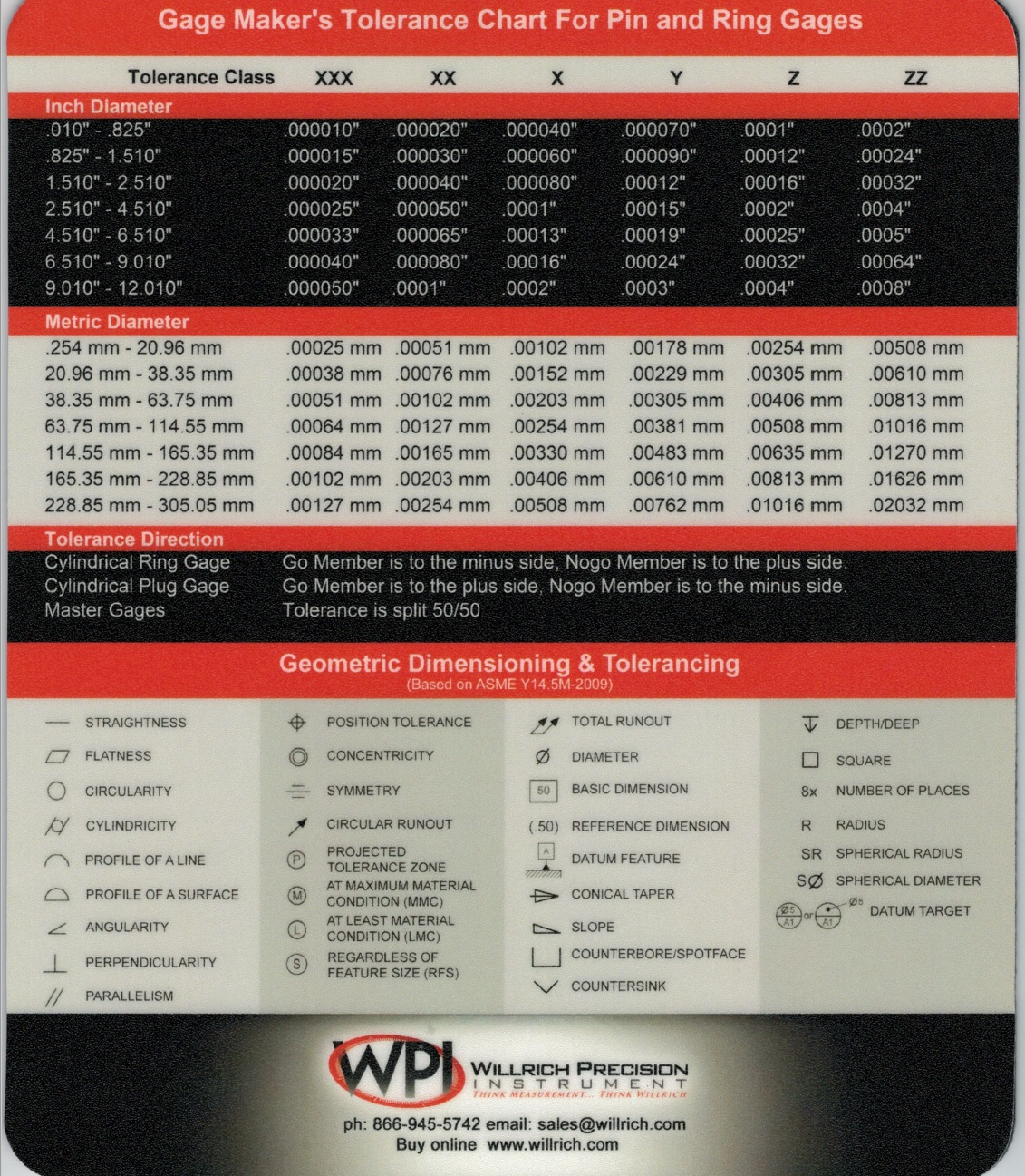# Understanding Millionths of an Inch

1.0000″=One Inch
.1000″=One Hundred Thousandths
.010″= Ten Thousandths
.001″= One Thousandth
.0001″=One Tenth (One Ten thousandth)
.00001″=Ten Millionths
.000001″=One Millionth
.000001″ = 1 Microinch
.0002″ = 200 Millionths Micro inches and Millionths are interchangeable
Inches X 25.4 = millimeters
Millimeters divided by 25.4 = inches
Microinches X .0254 = micrometers
Micrometers divided by .0254 = microinches
39.37 microinches =.00003937 inches = 1 micrometer (micron)
.001 millimeters =1 micrometer
.0254 micrometers =.0000254 millimeters
1 micron= 40 millionths of an inchFILL IN FORM BELOW TO RECEIVE  FREE MOUSEPAD OF GAGE MAKERS TOLERANCE & GD&T SYMBOLS